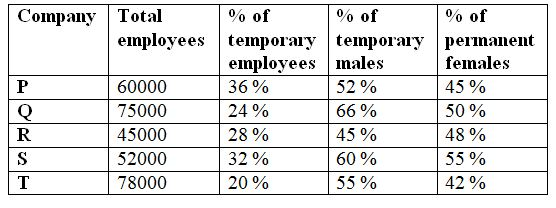# SBI Clerk prelims Quantitative Aptitude Questions 2019 (Day-08)

Quantitative aptitude is one of the most focusing subjects among banking aspirants.In SBI Clerk Prelims there are 35 Marks allotted for this section. The Marks itself shows how weightage has given for Quantitative aptitude section. The SBI Clerk 2019 exam is approaching very shortly. The exam tentatively scheduled in June 2019. Understand the importance of time and use it in the right way. As we know that SBI aptitude test questions are easy but difficult to solve within the given time limit. So practice the only solution to perform well at the time of examination. Keeping all the things in mind we are giving attention for SBI Clerk Prelims Quantitative Aptitude section. Here you can get SBI Clerk Prelims Quantitative Aptitude Questions and answer on a daily basis. SBI Clerk Prelims Quantitative Aptitude Quiz will enhance your sped and accuracy in a self-directed way.

[WpProQuiz 6252]

Directions (Q. 1 – 5): What approximate value should come in place of (?) in the following questions?

1) (113.27 + 854.73) ÷ 11 + (13.24)2 + (2.98/7) of 2519 – 27.92 = ?

a) 1310

b) 1450

c) 1560

d) 1240

e) 1670

2) (36.9/15) ÷ (74/132) × 24.72 = ? – (8.17)3

a) 745

b) 776

c) 575

d) 622

e) 718

3) 1401 ÷ 19.98 = ? + 425.78 – (311 ÷ 6 × 20.2)

a) 750

b) 520

c) 840

d) 430

e) 680

4) 36 % of 149 + (5/11) of 2859 – 30 % of 699 = ?

a) 1144

b) 1850

c) 1060

d) 2520

e) 4825

5) 15 11/12 % of 298 + (3/11) of 51644 + (14.21)2 = ?

a) 15120

b) 18560

c) 11440

d) 12750

e) 14330

Directions (Q. 6 – 10): Study the following information carefully and answer the given questions.

The following table shows the total employees working in 5 different companies and the percentage of permanent and temporary employees and also the percentage of permanent females and temporary males also given here.6) Find the difference between the total number of temporary females working in company P and S together to that of total number of permanent males working in company R and T together?

a) 36016

b) 32184

c) 28572

d) 38430

e) None of these

7) Find the ratio between the total number of temporary employees in company Q and R together to that of total number of permanent employees in company P and S together?

a) 152 : 247

b) 28 : 75

c) 379 : 621

d) 765 : 1844

e) None of these

8) Total number of temporary males working in company P is approximately what percentage of total number of permanent females working in company S?

a) 72 %

b) 58 %

c) 44 %

d) 36 %

e) 90 %

9) Find the difference between the average number of permanent female employees in company P and Q together to that of the average number of temporary female employees in company Q and T together?

a) 20740

b) 18450

c) 16320

d) 19580

e) None of these

10) Total number of employees working in company P and T together is approximately what percentage more/less than the total number of employees working in company Q and S together?

a) 10 % more

b) 20 % less

c) 5 % more

d) 15 % more

e) 10 % less

Directions (Q. 1 – 5):

(113.27 + 854.73) ÷ 11 + 132 + (3/7)*2520 – 28 = x

x = (968/11) + 169 + 1080 – 28

x = 88 + 169 + 1080 – 28 = 1309

x = 1310

(37/15)*(132/74)*25 = x – 83

110 + 512 = x

x = 622

(1400/20) = x + 426 – (312/6)*20

70 – 426 + 1040 = x

X = 684 = 680

(36/100)*150 + (5/11)*2860 – (30/100)*700 = x

x = 54 + 1300 – 210 = 1144

16 % of 300 + (3/11) * 51645 + 142 = x

(16/100)*300 + (3/11) * 51645 + 196 = x

48 + 14085 + 196 = x

X = 14329 = 14330

Directions (Q. 6 – 10):

The total number of temporary females working in company P and S together

= > 60000*(36/100)*(48/100) + 52000*(32/100)*(40/100)

= > 10368 + 6656 = 17024

The total number of permanent males working in company R and T together

= > 45000*(72/100)*(52/100) + 78000*(80/100)*(58/100)

= > 16848 + 36192 = 53040

Required difference = 53040 – 17024 = 36016

The total number of temporary employees in company Q and R together

= > 75000*(24/100) + 45000*(28/100)

= > 18000 + 12600 = 30600

The total number of permanent employees in company P and S together

= > 60000*(64/100) + 52000*(68/100)

= > 38400 + 35360 = 73760

Required ratio = 30600 : 73760 = 765 : 1844

Total number of temporary males working in company P

= > 60000*(36/100)*(52/100) = 11232

Total number of permanent females working in company S

= > 52000*(68/100)*(55/100) = 19448

Required % = (11232/19448)*100 = 58 %

The average number of permanent female employees in company P and Q together

= > [60000*(64/100)*(45/100) + 75000*(76/100)*(50/100)]/2

= > [17280 + 28500]/2 = 22890

The average number of temporary female employees in company Q and T together

= > [75000*(24/100)*(34/100) + 78000*(20/100)*(45/100)]/2

= > (6120 + 7020)/2 = 6570

Required difference = 22890 – 6570 = 16320

Total number of employees working in company P and T together

= > 60000 + 78000 = 138000

Total number of employees working in company Q and S together

= > 75000 + 52000 = 127000

Required % = ((138000 – 127000) /127000)*100 = 8.66 % = 10 %

Related Articles:

SBI Clerk prelims Quantitative Aptitude Questions 2019 (Day-07)

SBI Clerk prelims Quantitative Aptitude Questions 2019 (Day-06)

SBI Clerk prelims Quantitative Aptitude Questions 2019 (Day-05)